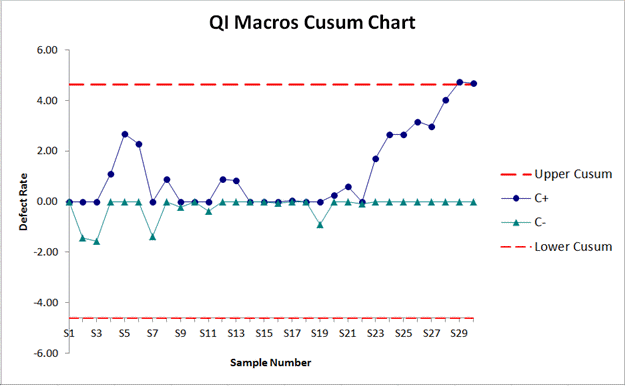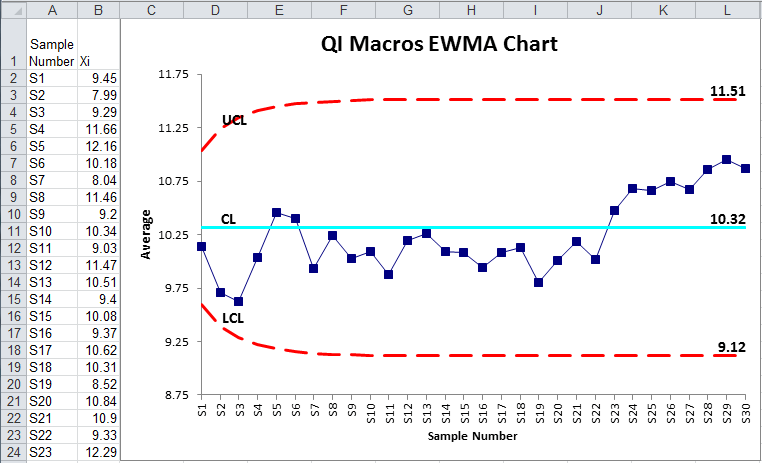# Trying to Create Cusum Charts in Excel?

## QI Macros can create cusum charts for you!

#### Draw a CUSUM Chart using QI Macros:

2. Select Control Charts (SPC) > Special> CUSUM from QI Macros menu.
3. QI Macros will do the math and draw the graph for you.

### Cumulative Sum Charts Can Detect Small Shifts in a Process

Cusum charts can detect process shifts more rapidly than the XmR or XbarR. If a trend develops, it's evidence that the process has shifted. In addition to a Cusum chart, you might consider an EWMA chart.

### Example of a Cusum Chart Created by QI Macros Add-in for Excel### A Cusum Chart consists of:

• A target which is either provided by you or calculated as the mean of your data.
• Upper Cusum Limit and Lower Cusum Limit lines.
• C+ and C- lines which represent the cumulative sum of deviations of successive sample means from a target. Use these lines to determine if your process is shifting.

#### Default values calculated in the Cusum Chart:

k (slack) = 1/2 of your StDev
(this is an estimated value, but can be changed)

FIR (Fast Initial Response) = 0
(This feature can be used to set an initial "head start" value to improve detection of an out-of-control condition at the start of the chart. If the process is in control, this initial head start has little effect; if the process is out of control, a signal will be given faster with a non-zero value than with a zero value)

h (threshold value or decision interval) = 4
(this determines how many StDev from the mean the Cusum Limits are set)

NOTE: Using h=4 or h=5 and k=.5 will generally provide a cusum that has good ARL properties (per Montgomery SPC 4th ed.)

### Compare a Cusum Chart with an EWMA Chart

##### Cusum Chart##### EWMA Chart### Compare a Cusum Chart with an Individual Moving Range Chart

##### Cusum Chart##### Individual Moving Range Chart